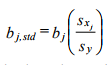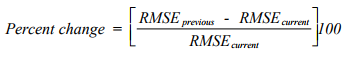# Stepwise Regression

Rating: 4

10171
1. Share:

In this article, we will discuss on a stepwise regression model which is one of the regression models which is used in the industry. Further, the stepwise regression model is explained with the help of a formula by taking an example.

## What is Stepwise Regression?

Stepwise regression is a type of regression technique that builds a model by adding or removing the predictor variables, generally via a series of T-tests or F-tests.

The variables, which need to be added or removed are chosen based on the test statistics of the coefficients estimated. Unlike other regression models, stepwise regression needs proper attention and only a skilled researcher who is familiar with statistical testing should perform it.

If you would like to become an SPSS Certified professional, then visit Mindmajix - A Global online training platform:" SPSS Certification Training Course ". This course will help you to achieve excellence in this domain.

So no let’s understand the working pricing of Stepwise regression and what are the points that we need to consider:

There are mainly two ways to perform stepwise regression. These are as followed:

1. A test is started with all available predictor variables

2. A test is started with no predictor variables

### What is Backward Elimination?

Backward elimination is also called as Step down elimination.

The first type of test which the software performs is called as the “Backward (Step-down) Elimination” where one variable is deleted at a time during the regression model’s progress. If you have a modest no. of predictor variables and you want to eliminate a few of them, you can use this test method. As the regression model progresses, the variable with the lowest F-to-remove statistic is deleted at each step from the model.

This F-to-remove statistic is calculated as followed.

• Calculating the t-statistic for the estimated coefficient of every variable in the model
• Squaring the t-statistic to create the F-to-remove statistic

### What is Forward Selection?

Forward selection is also called as Step-up selection.

In the second type of test, which is also called the “Forward (Step-up) Selection”, variables are added one at a time as the regression model progresses. This method is generally used when there is a large set of predictor variables. The same steps as above are followed to create the F-to-add statistic, except that the statistic is calculated for each variable not in the model. So in this process, the variable with the highest F-to-add statistic will be getting added to the model.

So understanding the two types of tests combined will help the individual to carry on with stepwise regression.

Further, the two above tests can also be combined to perform stepwise regression where the test will happen at each step for the variables to be included or excluded. This test is also called “Bidirectional (Stepwise) Elimination”. This can also be done by specifying a minimum change in the root mean square error instead of using probabilities to add and remove, this process is called “Min MSE”.## Stepwise regression formula

If you standardize each dependent and independent variable that is you subtract the mean and divide by the standard deviation of a variable, you will get the standardized regression coefficients. Below is the formula that illustrates it:Where Sy and Sxj are the standard deviations for the dependent variable and the corresponding jth independent variable

The percentage change in the square root of mean square error, which will occur if the specified variables are added to, or deleted from the model, is called as RMSE. This value is used by the Min MSE method. This percentage change in Root Mean Square Error (RMSE) is calculated as below:Example:

Stepwise regression is used to determine one or a few causal factors or dependent variables when you have a large number of dependent variables. This method is mostly used in feedback surveys where the participants are asked to provide feedback on a particular question like why do they like the service. Their responses are then fed into the stepwise regression method and the responses with the lowest F-to-remove values are eliminated. By repeating the regression by eliminating one response at a time we can identify the most relevant answers.

### Conclusion:

So today we understood how stepwise regression is applied in the industry and what all it takes for the organizations to come to a conclusion. With the help of this regression, one would be able to gather quality inputs from the feedback surveys and will be able to deliver outputs as per the organization’s needs.

### Related Regression Articles:Course Schedule
NameDates
IBM SPSS TrainingFeb 07 to Feb 22
IBM SPSS TrainingFeb 11 to Feb 26
IBM SPSS TrainingFeb 14 to Mar 01
IBM SPSS TrainingFeb 18 to Mar 05
Last updated: 06 February 2023